# NCERT Solutions For Class 11 Math Chapter – 8 Exercise – 8.2

NCERT  Solutions For Class 11 Math Chapter – 8 Exercise – 8.2

Find the coefficient of

1. x5 in (x + 3)8

Solution:

The general term Tr+1 in the binomial expansion is given by Tr+1 = r an-r br

Here x5 is the Tr+1 term so a= x, b = 3 and n =8

Tr+1 = 8Cr x8-r 3r…………… (i)

For finding out x5

We have to equate x5= x8-r

⇒ r= 3

Putting value of r in (i) we get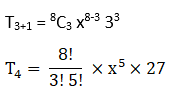= 1512 x5

Hence the coefficient of x5= 1512

2. a5b7 in (a – 2b)12 .

Solution:

The general term Tr+1 in the binomial expansion is given by Tr+1 = r an-r br

Here

a = a, b = -2b & n =12

Substituting the values, we get

Tr+1 = 12Cr a12-r (-2b)r………. (i)

To find a5

We equate a12-r =a5

r = 7

Putting r = 7 in (i)

T8 = 12C7 a5 (-2b)7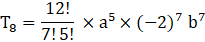= -101376 a5 b7

Hence the coefficient of a5b7= -101376

Write the general term in the expansion of

3. (x2 – y)6

Solution:

The general term Tr+1 in the binomial expansion is given by

Tr+1 = r an-r br…….. (i)

Here a = x2 , n = 6 and b = -y

Putting values in (i)

Tr+1 = 6Cr x 2(6-r) (-1)r yr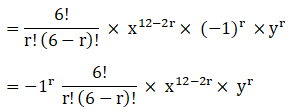= -1r 6c.x12 – 2r. yr

4. (x2 – y x)12, x ≠ 0.

Solution:

The general term Tr+1 in the binomial expansion is given by Tr+1 = r an-r br

Here n = 12, a= x2 and b = -y x

Substituting the values we get

Tn+1 =12Cr × x2(12-r) (-1)r yr xr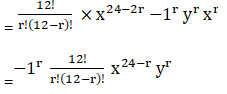= -1r 12c.x24 –2r. yr

5. Find the 4th term in the expansion of (x – 2y)12.

Solution:

The general term Tr+1 in the binomial expansion is given by Tr+1 = r an-r br

Here a= x, n =12, r= 3 and b = -2y

By substituting the values we get

T4 = 12C3 x9 (-2y)3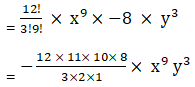= -1760 x9 y3

6. Find the 13th term in the expansion of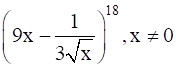Solution: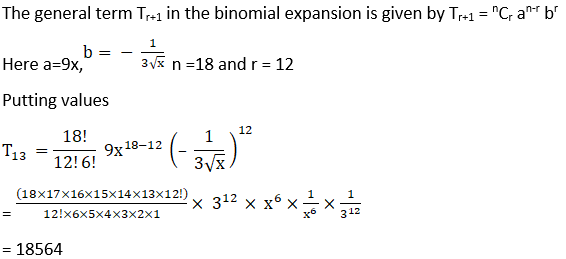Find the middle terms in the expansions of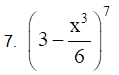Solution: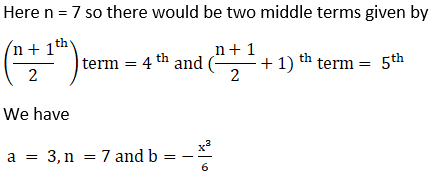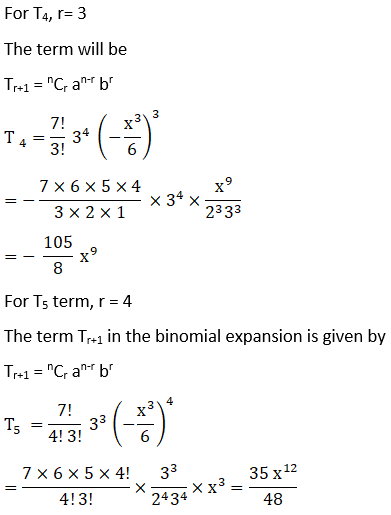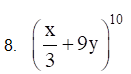Solution: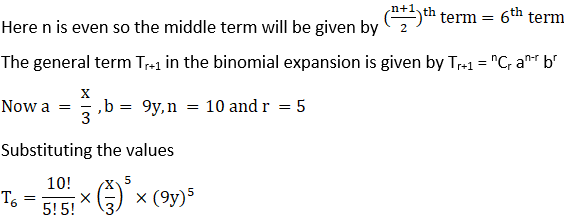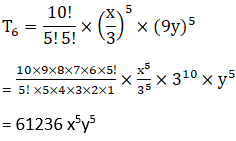9. In the expansion of (1 + a)m+n, prove that coefficients of am and an are equal.

Solution:

We know that the general term Tr+1 in the binomial expansion is given by Tr+1 = nCr an-r br

Here n= m+n, a = 1 and b= a

Substituting the values in the general form

Tr+1 = m+n Cr 1m+n-r ar

m+n Cr ar…………. (i)

Now we have that the general term for the expression is,

Tr+1 =  m+n Cr ar

Now, For coefficient of am

Tm+1 =  m+n Cm am

Hence, for coefficient of am, value of r = m

So, the coefficient is m+n m

Similarly, Coefficient of an is m+n n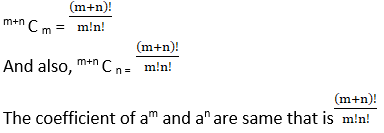10. The coefficients of the (r – 1)th, rth and (r + 1)th terms in the expansion of (x + 1)n are in the ratio 1 : 3 : 5. Find n and r.

Solution:

The general term Tr+1 in the binomial expansion is given by Tr+1 = nCr an-r br

Here the binomial is (1+x)n with a = 1 , b = x and n = n

The (r+1)th term is given by

T(r+1) = nCr 1n-r xr

T(r+1) = nCr xr

The coefficient of (r+1)th term is nCr

The rth term is given by (r-1)th term

T(r+1-1) = nCr-1 xr-1

Tr = nCr-1 xr-1

∴ the coefficient of rth term is nCr-1

For (r-1)th term we will take (r-2)th term

Tr-2+1 = nCr-2 xr-2

Tr-1 = nCr-2 xr-2

∴ the coefficient of (r-1)th term is nCr-2

Given that the coefficient of (r-1)th, rth and r+1th term are in ratio 1:3:5

Therefore,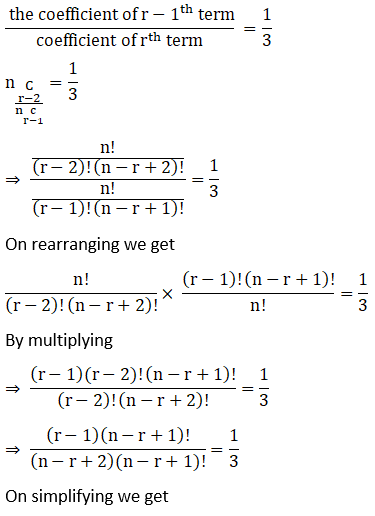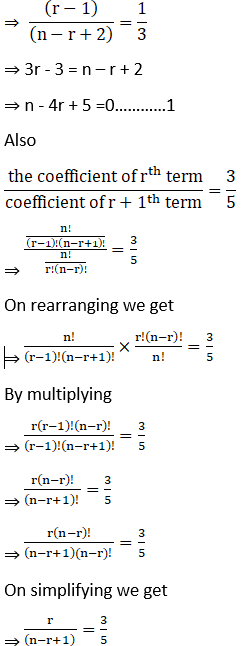⇒ 5r = 3n – 3r + 3

⇒ 8r – 3n – 3 =0………….2

We have 1 and 2 as

n – 4r ± 5 =0…………1

8r – 3n – 3 =0…………….2

Multiplying equation 1 by number 2

2n -8r +10 =0……………….3

2n -8r +10 =0

-3n – 8r – 3 =0

⇒ -n = -7

n =7 and r = 3

11. Prove that the coefficient of xn in the expansion of (1 + x)2n is twice the coefficient of xn in the expansion of (1 + x)2n – 1.

Solution:

The general term Tr+1 in the binomial expansion is given by Tr+1 = nCr an-r br

The general term for binomial (1+x)2n is

Tr+1 = 2nCr xr …………………..1

To find the coefficient of xn

r = n

Tn+1 = 2nCn xn

The coefficient of xn = 2nCn

The general term for binomial (1+x)2n-1 is

Tr+1 = 2n-1Cr xr

To find the coefficient of xn

Putting n = r

Tr+1 = 2n-1Cr xn

The coefficient of xn = 2n-1Cn

We have to prove

Coefficient of xn in (1+x)2n = 2 coefficient of xn in (1+x)2n-1

Consider LHS = 2nCn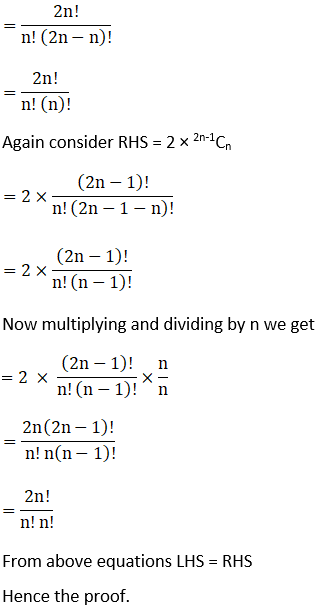12. Find a positive value of m for which the coefficient of x2 in the expansion (1 + x)m is 6.

Solution:

The general term Tr+1 in the binomial expansion is given by Tr+1 = nCr an-r br

Here a = 1, b = x and n = m

Putting the value

Tr+1 = Cr 1m-r xr

Cr xr

We need coefficient of x2

∴ putting r = 2

T2+1 = mC2 x2

The coefficient of x2 = mC2

Given that coefficient of x2 = mC2 = 6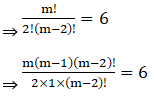⇒ m (m – 1) = 12

⇒ m2– m – 12 =0

⇒ m2– 4m + 3m – 12 =0

⇒ m (m – 4) + 3 (m – 4) = 0

⇒ (m+3) (m – 4) = 0

⇒ m = – 3, 4

We need positive value of m so m = 4